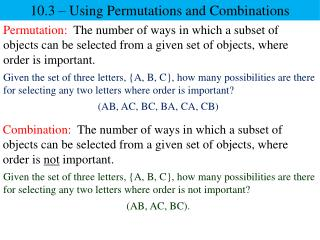# 10.3 – Using Permutations and Combinations - PowerPoint PPT PresentationDownload Presentation10.3 – Using Permutations and Combinations

10.3 – Using Permutations and CombinationsDownload Presentation## 10.3 – Using Permutations and Combinations

- - - - - - - - - - - - - - - - - - - - - - - - - - - E N D - - - - - - - - - - - - - - - - - - - - - - - - - - -
##### Presentation Transcript

1. 10.3 – Using Permutations and Combinations Permutation: The number of ways in which a subset of objects can be selected from a given set of objects, where order is important. Given the set of three letters, {A, B, C}, how many possibilities are there for selecting any two letters where order is important? (AB, AC, BC, BA, CA, CB) Combination: The number of ways in which a subset of objects can be selected from a given set of objects, where order is not important. Given the set of three letters, {A, B, C}, how many possibilities are there for selecting any two letters where order is not important? (AB, AC, BC).

2. 10.3 – Using Permutations and Combinations Factorial Formula for Permutations Factorial Formula for Combinations

3. 10.3 – Using Permutations and Combinations Evaluate each problem. d) 6C6 b) 5C3 c) 6P6 a) 5P3 543 60 720 10 1

4. 10.3 – Using Permutations and Combinations How many ways can you select two letters followed by three digits for an ID if repeats are not allowed? Two parts: 1. Determine the set of two letters. 2. Determine the set of three digits. 26P2 10P3 2625 1098 650 720 650720 468,000

5. 10.3 – Using Permutations and Combinations A common form of poker involves hands (sets) of five cards each, dealt from a deck consisting of 52 different cards. How many different 5-card hands are possible? Hint: Repetitions are not allowed and order is not important. 52C5 2,598,960 5-card hands

6. 10.3 – Using Permutations and Combinations Find the number of different subsets of size 3 in the set: {m, a, t, h, r, o, c, k, s}. Find the number of arrangements of size 3 in the set: {m, a, t, h, r, o, c, k, s}. 9C3 9P3 987 504 arrangements 84 Different subsets

7. 10.3 – Using Permutations and Combinations Guidelines on Which Method to Use